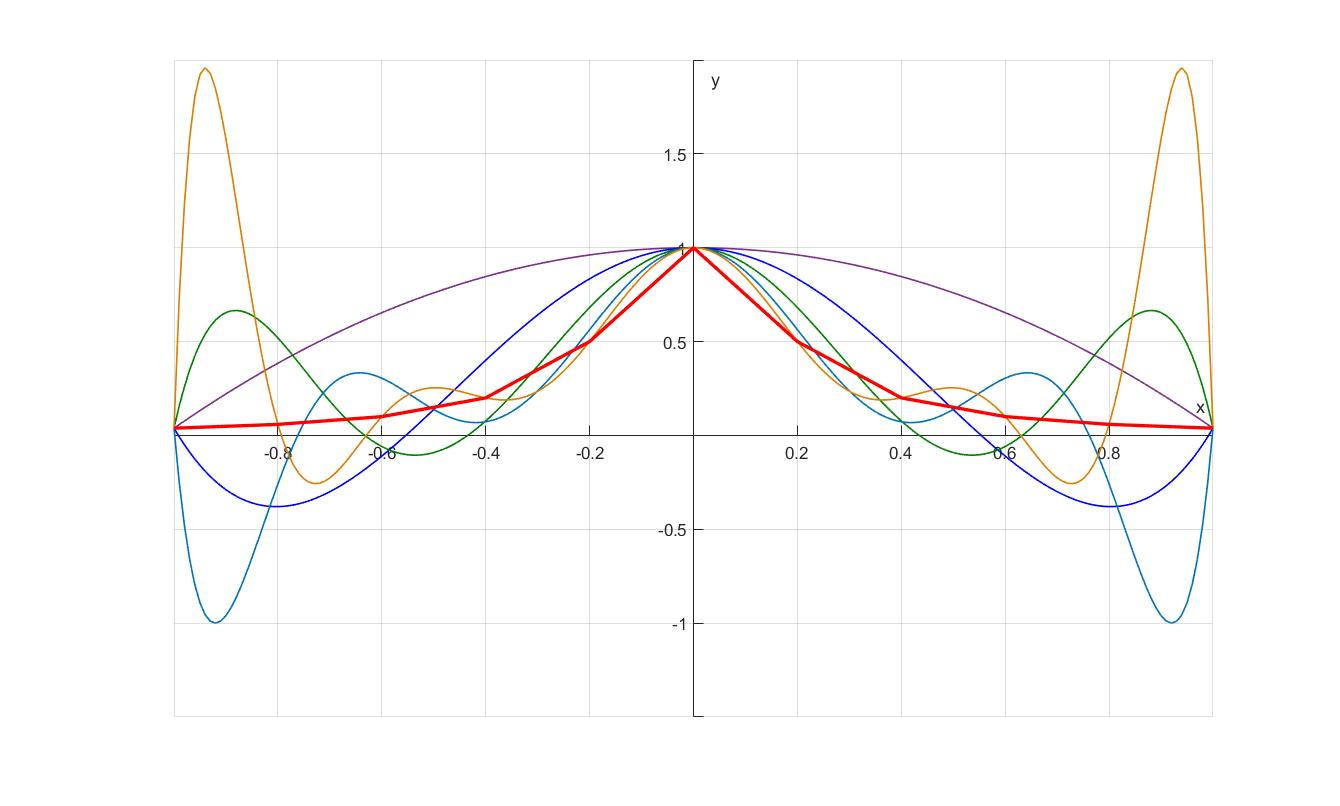# 逼近理论缘起

• 存在性: 逼近函数是否存在?
• 具体形式: 如果存在, 那么具体如何构造呢?
• 唯一性: 满足条件的逼近函数是否是唯一的?

# 函数逼近理论中常用概念引入

• $g$ 到 $f$ 的距离为:
$$\Delta(g) = \sup_{x\in X}|f-g|.$$
• 定义在 $X$ 上的有界函数 $f$ 的连续模 (Modulus of Continuity):
$$\omega(\delta) = \sup_{\small{\begin{array}{c} \mid x_1-x_2 \mid < \delta \\ x_1,x_2 \in E \end{array}}} |f(x_1)-f(x_2)|\quad(\delta>0).$$
• 不超过 $n$ 次的多项式构成的函数类用 $P_{n}$ 表示. 定义
$$E_n(f) = \inf_{p_n\in P_n}\Delta(p_n).$$
• 若有多项式 $p^{\star}_n\in P_n$ 满足 $\Delta(p^{\star} _{n}) = E _n(f)$, 则其称为函数 $f$ 的**$n$ 次最佳逼近多项式**.

# 魏尔斯特拉斯(Weierstrass)逼近定理的 Bernstein 证明

Weierstrass Approximation Theorem】 令 $f$ 为定义在闭区间 $[a,b]$ 上的函数. 那么, $\forall \varepsilon>0$, 存在多项式 $p$, 使得 $|f-p|<\varepsilon$.

【伯恩斯坦 (Bernstein) 多项式】 设 $f$ 为定义在 $[0, 1]$ 上的有界函数. 以下多项式序列称为 $f$ 的 Bernstein 多项式:
$$\left(B_n(f)\right)(x) = \sum^{n} _{k=0}{f\left(\frac{k}{n}\right)C^{k} _{n} x^k (1-x)^{n-k}},\quad 0\leqslant x \leqslant 1.$$

$$f_0(x) = 1,\quad f_1(x) = x,\quad f_2 = x^2.$$

【引理】

• a) $B_n(f_0) = f_0$, $B_n(f_1) = f_1$;
• b) $B_n(f_2) = (1-\frac{1}{n})f_2 +\frac{1}{n}f_1$, 并且 $B_{n}(f_2)\rightrightarrows f_2$;
• c) $\sum^{n} _{k=0}{\left(\frac{k}{n}-x\right)^2 C^{k} _{n} x^k (1-x)^{n-k}} = x(1-x)/n \leqslant \frac{1}{4n},\quad \text{if}\quad 0\leqslant x \leqslant 1.$
• d) 给定 $\delta>0$ 以及 $0\leqslant x \leqslant 1$, 用 $D$ 表示 $\{1,\cdots, n\}$ 中使得 $|k/n -x|>\delta$ 的那些 $k$ 构成的集合. 那么$\sum^{n} _{k\in D}{C^{k} _{n} x^k (1-x)^{n-k}}\leqslant \frac{1}{4n\delta ^2}$.

【证明】
a) 由二项式展开, 立刻有 $B_n(f_0) = [x + (1-x)]^n = 1 = f_0$; 而
\begin{array}{cl}
B_n(f_1) &=& \sum^{n}\limits _{k=0} {\frac{k}{n}C^{k} _{n} x^k (1-x)^{n-k}} \\
&=& \sum^{n}\limits _{k=0}{\frac{(n-1)!}{(n-k)!(k-1)!} x^k (1-x)^{n-k}} \\
&=& x \sum^{n}\limits _{k=0}{\frac{(n-1)!}{(n-k)!(k-1)!} x^{k-1} (1-x)^{n-k}} \\
&=& x [x + (1-x)]^{n-1} = x.
\end{array}

b) 类似的, 依旧通过二项式展开定理证明:
\begin{array}{cl}
B_n(f_2) &=& \sum^{n}\limits _{k=0} {\left(\frac{k}{n}\right)^2C^{k} _{n} x^k (1-x)^{n-k}} \\
&=& \sum^{n}\limits _{k=0}{\frac{k}{n}\frac{(n-1)!}{(n-k)!(k-1)!} x^k (1-x)^{n-k}} \\
&=& \sum^{n}\limits _{k=0}{\left(\frac{k-1}{n-1}\frac{n-1}{n}+\frac{1}{n}\right) \frac{(n-1)!}{(n-k)!(k-1)!} x^k (1-x)^{n-k}}\\
&=& \sum^{n}\limits _{k=0}{\left(\frac{k-1}{n-1}\right)\left(\frac{n-1}{n}\right) \frac{(n-1)!}{(n-k)!(k-1)!} x^k (1-x)^{n-k}} + \frac{x}{n} \\
&=& \left(\frac{n-1}{n}\right)x^2 [x + (1-x)]^{n-2} + \frac{1}{n}x\\
&=& (1-\frac{1}{n})f_2 +\frac{1}{n}f_1.
\end{array}显然, $n\rightarrow\infty$时有 $B_n(f_2)\rightarrow f_2$, 而且这个收敛是一致的.

c) 展开 $\left(\frac{k}{n}-x\right)^2 = \left(\frac{k}{n}\right)^2 - 2\left(\frac{k}{n}\right) x + x^2$. 利用 a) 和 b) 的结果, 注意 $0\leqslant x \leqslant 1$, 于是得到
\begin{array}{cl}
& \sum^{n} _{k=0}{\left(\frac{k}{n}-x\right)^2 C^{k} _{n} x^k (1-x)^{n-k}} \\
= & (1-\frac{1}{n})x^2 +\frac{1}{n}x - 2x^2 + x^2\\
= & \frac{1}{n}(x^2-x) \leqslant \frac{1}{4n}.
\end{array}

d) 注意到 $|k/n -x|>\delta$, 则 $(k/n -x)^2 /\delta^2 >1$, 故
\begin{array}{cl}
& \sum^{n} _{k\in D}{C^{k} _{n} x^k (1-x)^{n-k}} \\
\leqslant & \frac{1}{\delta^2}\sum^{n} _{k=0}{\left(\frac{k}{n}-x\right)^2 C^{k} _{n} x^k (1-x)^{n-k}}\leqslant \frac{1}{4n\delta^2}.
\end{array}

\begin{array}{cl}
|f- B_n(f)| &=& \left| f(x) - \sum^{n} _{k=0}{f\left(\frac{k}{n}\right)C^{k} _{n} x^k (1-x)^{n-k}} \right| \\
&=& \left| \sum^{n} _{k=0}{\left(f(x) - f\left(\frac{k}{n}\right)\right)C^{k} _{n} x^k (1-x)^{n-k}} \right| \\
&\leqslant& \sum^{n} _{k=0}{\left|f(x) - f\left(\frac{k}{n}\right)\right|C^{k} _{n} x^k (1-x)^{n-k}}.
\end{array} 由于 $f$ 有界, 设为 $M$; 同时$f$ 又是区间上的连续函数 $\forall \varepsilon/2 > 0$, $\exists\delta > 0$, $\forall x, x_1 \in [1 ,0]$, $|x-x_1|<\delta$ 有 $|f(x)-f(x_1)|<\varepsilon$. 于是按照上述引理的 d) 有:
\begin{array}{cl}
& \sum^{n} _{k=0}{\left|f(x) - f\left(\frac{k}{n}\right)\right|C^{k} _{n} x^k (1-x)^{n-k}} \\
=& \sum _{k\notin D}{\left|f(x) - f\left(\frac{k}{n}\right)\right|C^{k} _{n} x^k (1-x)^{n-k}}\\
&+\sum _{k \in D}{\left|f(x) - f\left(\frac{k}{n}\right)\right|C^{k} _{n} x^k (1-x)^{n-k}}\\
\leqslant & \frac{\varepsilon}{2} + 2M\frac{1}{4n\delta^2}.
\end{array}

Bernstein 通过这一构造, 完成了对于魏尔斯特拉斯逼近定理的证明. 这个证明的重要意义在于其实实在在的构造出了一个一致收敛的多项式函数序列, 为寻找函数 $f$ 的逼近多项式提供了一个便捷的显式表达. 但这会是最佳的逼近多项式吗? 事实是不一定. 以前述 $f(x) = x^2$ 为例, 显然, 其最佳逼近多项式就是自身, 但是其 Bernstein 多项式为 $(1-1/n)x^2 + (1/n)x$. 到现在为止, 我们只解决了第一个, 关于逼近多项式函数存在的问题, 并给出了一种可能的构造这样的多项式的方法. 至于最佳逼近, 以及逼近函数唯一性的问题, 则暂时留待下次再谈.

Zengfk

2019-08-11

2021-03-09